SSC  >  Test: Soil Mechanics

# Test: Soil Mechanics

Test Description

## 20 Questions MCQ Test Mock Test Series of SSC JE Civil Engineering | Test: Soil Mechanics

Test: Soil Mechanics for SSC 2023 is part of Mock Test Series of SSC JE Civil Engineering preparation. The Test: Soil Mechanics questions and answers have been prepared according to the SSC exam syllabus.The Test: Soil Mechanics MCQs are made for SSC 2023 Exam. Find important definitions, questions, notes, meanings, examples, exercises, MCQs and online tests for Test: Soil Mechanics below.
Solutions of Test: Soil Mechanics questions in English are available as part of our Mock Test Series of SSC JE Civil Engineering for SSC & Test: Soil Mechanics solutions in Hindi for Mock Test Series of SSC JE Civil Engineering course. Download more important topics, notes, lectures and mock test series for SSC Exam by signing up for free. Attempt Test: Soil Mechanics | 20 questions in 12 minutes | Mock test for SSC preparation | Free important questions MCQ to study Mock Test Series of SSC JE Civil Engineering for SSC Exam | Download free PDF with solutions
 1 Crore+ students have signed up on EduRev. Have you?
Test: Soil Mechanics - Question 1

### In Boussinesq’s solution to the problem of stress distribution, all the following assumptions are made, except:

Detailed Solution for Test: Soil Mechanics - Question 1

Assumptions made in Boussinesq’s Theory are:

1. Soil is homogeneous, semi-infinite, elastic and isotropic.

2. Hooke’s law is valid.

3. Any change in the volume of soil due to application of load is neglected.

4. Distribution of stresses along the vertical axis is symmetrical.

5. Self-weight of soil is neglected.

Test: Soil Mechanics - Question 2

### Spring analogy in soil mechanics is used for explaining which of the following phenomenon?

Detailed Solution for Test: Soil Mechanics - Question 2

Terzaghi in 1922 demonstrated a model (Spring-piston model) to study the mechanism of consolidation in clay. The model consists of a cylindrical vessel with the series of pistons separated by springs. The space between the springs is filled with water and the pistons are perforated so as to allow through communication to water. Piezometers are inserted at the centres of each of the compartments to measure the pressure heads due to excess pore water pressures. The springs surrounded by water represent the saturated soil. The springs represents the soil skeleton and the water in the vessel represents the water filling the voids in the soil.

Test: Soil Mechanics - Question 3

### The term _________ on soil is used to indicate the maximum pressure which can be exerted on soil while taking into account shear failure, settlement and the ability of structure to resist settlement.

Detailed Solution for Test: Soil Mechanics - Question 3

The value of Allowable Bearing Pressure on soil depends on the nature of ground and the type of building. The maximum safe bearing capacity of soil is generally more than the allowable bearing pressure on soil.

Test: Soil Mechanics - Question 4

If the coefficient of uniformity of a soil is 16 and the coefficient of curvature is 1, then what is the ratio D60/D30?

Detailed Solution for Test: Soil Mechanics - Question 4

Cu = 16

Cc = 1

16=Cu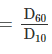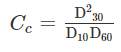D60 = 16 D10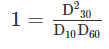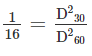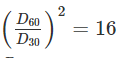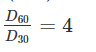Test: Soil Mechanics - Question 5

The shear test in which full consolidation occurs and excess pore pressure is build up during shearing stage is known as:

Detailed Solution for Test: Soil Mechanics - Question 5

In first stage (application of cell pressure stage) soil will consolidate because drainage valve is open and pore water is drain out of soil leads to consolidation, In second stage (Shearing stage) drainage valves are closed leads to development of excess pore water pressure, this implies first stage is consolidated(C) and second stage is undrained(U). So the test is CU test.

Test: Soil Mechanics - Question 6

The permeability of a soil varies:

Detailed Solution for Test: Soil Mechanics - Question 6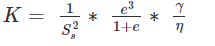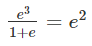Where Ss = specific surface area =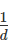Where d = grain size

So, permeability is directly proportional to the square of grain size.

Test: Soil Mechanics - Question 7

A soil mass has a void ratio of 0.67? How much water (in litres) will be required to fully saturate 1 m3 of the soil?

Detailed Solution for Test: Soil Mechanics - Question 7

e = 0.68

Volume of soil = 1m3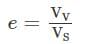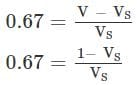0.67 VS = 1 – VS

1.67 VS = 1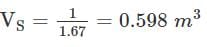VV = V – VS = 1 – 0.598 = 0.402 m3

Initially we assume that soil is completely dry

VV = Vwater required to fully saturate the soil mass

Vwater = 0.402 × 1000 = 402 litres

Test: Soil Mechanics - Question 8

If the fineness modulus of sand is 3, then the sand is graded as

Detailed Solution for Test: Soil Mechanics - Question 8

Fineness modulus of sand:

1. Fine sand: 2.2 to 2.6

2. Medium sand: 2.6 to 2.9

3. Coarse sand: 2.9 to 3.2

So, Fineness modulus 3 corresponds to coarse sand.

Test: Soil Mechanics - Question 9

Find the shrinkage ration of a soil sample whose plastic limit and liquid limit values are 30% and 42%, respectively, and the values of percentage of volume change from liquid limit to dry state and plastic limit to dry state are 35% and 22%, respectively, of dry volume.

Detailed Solution for Test: Soil Mechanics - Question 9

corresponding change in the water content of the soil above shrinkage limit.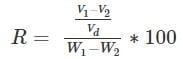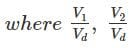are percentage change of volume from liquid limit to dry state and plastic limit to dry state respectively

W1 and W2 are corresponding water content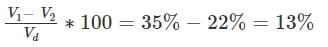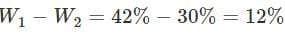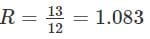Test: Soil Mechanics - Question 10

In vane shear test, the torque rod is rotated at a uniform speed of:

Detailed Solution for Test: Soil Mechanics - Question 10

In Vane shear test, the vane is pushed in to the soil and torque is applied manually which is measured by a calibrated torsional spring. The rate of rotation of vane is nearly 6per minute or 0.1o per second.

Test: Soil Mechanics - Question 11

Which of the following shows the CORRECT order of increasing surface areas of the given soil?

Detailed Solution for Test: Soil Mechanics - Question 11

Specific Surface area is inversely proportional to grain size. Out of sand, silt, clay and colloids, Sand has highest grain size while colloids have least grain size.

Test: Soil Mechanics - Question 12

What is the assumption made about back of wall, in the Rankine’s theory of earth pressure?

Detailed Solution for Test: Soil Mechanics - Question 12

Assumptions given by Rankine are:

1. The soil is homogeneous and isotropic, which means c, φ and γ have the same values everywhere, and they have the same values in all directions at every point.

2. The most critical shear surface is a plane.

3. The ground surface is a plane.

4. The wall is infinitely long so that the problem may be analysed in only two dimensions.

5. The wall moves sufficiently to develop the active or passive condition.

6. The wall surface at the back is vertical and smooth.

Test: Soil Mechanics - Question 13

If in a soil mass, the product of degree of saturation (s) and void ratio (e) is 1. Then water content (ω) is

Detailed Solution for Test: Soil Mechanics - Question 13

Se = ωG

1 = ωG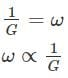Test: Soil Mechanics - Question 14

A soil has a bulk density of 22 kN/m3 and water content of 12%. The dry density of soil is

Detailed Solution for Test: Soil Mechanics - Question 14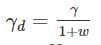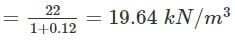Test: Soil Mechanics - Question 15

What is the ratio of discharge through the tube well to the discharge through the open well

Detailed Solution for Test: Soil Mechanics - Question 15

Discharge through the open well is in the range of (3-6) litres/sec while discharge through the tube well is in the range of (200 - 220) l/sec. So the approximate ratio is 50.

Test: Soil Mechanics - Question 16

The pile provided with one or more bulbs in its vertical shaft, is generally known as

Detailed Solution for Test: Soil Mechanics - Question 16

The pile provided with one or more bulbs in the vertical shaft is known as under reamed pile. This kind of pile is provided in case of expansive soil like black cotton soil.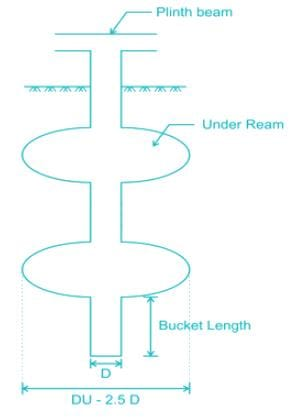Test: Soil Mechanics - Question 17

The angle of inclination of the plane at which the body begins to move down the plane, is called

Detailed Solution for Test: Soil Mechanics - Question 17

The angle of inclination of the plane at which the body begins to move down the plane, is called angle of repose.

Test: Soil Mechanics - Question 18

Match List I (Types of Soil) with List II (weathering agent) and select the answer option given below:-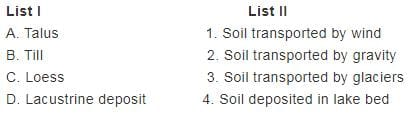Detailed Solution for Test: Soil Mechanics - Question 18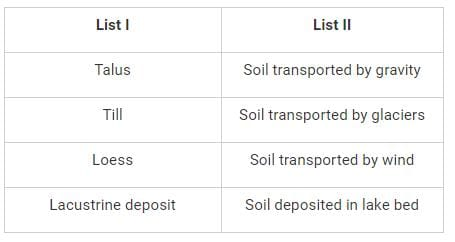Test: Soil Mechanics - Question 19

The maximum height of an unsupported vertical cut in a cohesive soil is given by ________

Detailed Solution for Test: Soil Mechanics - Question 19

Depth at which the earth pressure become zero in case of cohesive soil is given by expression,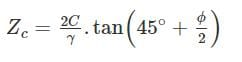So the depth of unsupported vertical cut is,Test: Soil Mechanics - Question 20

Shear strength of soil increases with increase in -

Detailed Solution for Test: Soil Mechanics - Question 20

Shearstrength,τ=C+σtanϕ

Where C = Cohesion

σ = Nomal stress

ϕ = Angle of internal friction.

So all the above options are correct. Shear strength will increase with increase in cohesion, angle of internal friction and normal stress on soil.

## Mock Test Series of SSC JE Civil Engineering

1 videos|1 docs|64 tests
 Use Code STAYHOME200 and get INR 200 additional OFF Use Coupon Code
Information about Test: Soil Mechanics Page
In this test you can find the Exam questions for Test: Soil Mechanics solved & explained in the simplest way possible. Besides giving Questions and answers for Test: Soil Mechanics , EduRev gives you an ample number of Online tests for practice

## Mock Test Series of SSC JE Civil Engineering

1 videos|1 docs|64 tests